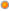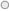# PowerPivot DAX function list with samples

User Rating: 4 / 5Note:
This is work in progress; please check later for more samples.

Logical DAX Functions

 Function Description Sample code IF(logical_test>, ,  value_if_false) Checks if a condition provided as the first argument is met. Returns one value if the condition is TRUE, and returns another value if the condition is FALSE. For table DimTime add calculation "Weekend Flag" =IF([EnglishDayNameOfWeek] = "Saturday" || [EnglishDayNameOfWeek] = "Sunday", "Weekend","Not Weekend") NOT() Changes FALSE to TRUE, or TRUE to FALSE. For table DimTime add calculation “Weekday Flag”: =NOT(IF([EnglishDayNameOfWeek] = "Saturday" || [EnglishDayNameOfWeek] = "Sunday", True(), False())) True() Returns logical value TRUE For table DimTime add calculation "Weekend Flag" =IF([EnglishDayNameOfWeek] = "Saturday" || [EnglishDayNameOfWeek] = "Sunday", True(), False()) False() Returns logical value FALSE For table DimTime add calculation "Weekend Flag" =IF([EnglishDayNameOfWeek] = "Saturday" || [EnglishDayNameOfWeek] = "Sunday", True(), False())

Filter and value DAX Functions

 Function Description Sample code ALL() Returns all the rows in a table, or all the values in a column, ignoring any filters that might have been applied. For table DimCustomer add calculation “Count ratio to ALL”: =COUNTROWS(RELATEDTABLE(FactInternetSales))/ COUNTROWS(ALL(FactInternetSales)) * 100 Note: This function makes more sense for pivot calculations   For Pivot table add calculated value field “Sales Amount Total Ratio” =SUMX(FactInternetSales,  FactInternetSales[SalesAmount]) / SUMX(ALL(FactInternetSales),  FactInternetSales[SalesAmount]) * 100 ALLEXCEPT(,column1>,,…) Overrides all context filters in the table except filters that have been applied to the specified columns. For Pivot table add calculated value field “Sales Amount Ratio”: =SUMX(FactInternetSales,  FactInternetSales[SalesAmount]) / SUMX(ALLEXCEPT(FactInternetSales,DimDate[FiscalYear]),  FactInternetSales[SalesAmount]) * 100 Note: while calculating total sales we consider values of attribute DimDate[FiscalYear] BLANK() Returns a blank. CALCULATE(,,…) Evaluates an expression in a context that is modified by the specified filters. For table DimCustomer add calculation “Distinct Order Cnt” to return distinct SalesOrderNumber count: =Calculate(COUNTROWS(Distinct(FactInternetSales[SalesOrderNumber]))) CALCULATETABLE( , , ,…) Evaluates a table expression in a context modified by filters. DISTINCT() Returns a one-column table that contains the distinct values from the specified column. For table DimCustomer add calculation “Distinct Order Cnt” to return distinct SalesOrderNumber count: =Calculate(COUNTROWS(Distinct(FactInternetSales[SalesOrderNumber]))) EARLIER(, ) Returns the current value of the specified column in an outer evaluation pass of the mentioned column. -(Optional) A positive number to the outer evaluation pass. EARLIEST() Returns the current value of the specified column in an outer evaluation pass of the mentioned column FILTER(,) Returns a table that represents a subset of another table or expression. For DimCustomer table add field “Discounted Order cnt”: =COUNTROWS(FILTER(RelatedTable(FactInternetSales), [PromotionKey]<>1)) Note when PromotionKey<>1, then there is a discount applied RELATED() Returns a related value from another table For table FactInternetSales add calculated column “Product Name”: =Related(DimProduct[EnglishProductName]) RELATEDTABLE() Follows an existing relationship, in either direction, and returns a table that contains all matching rows from the specified table. For table DimProduct ctInternetSales table sum order quantity and add result to each product line   =SUMX(RELATEDTABLE(FactInternetSales), FactInternetSales[OrderQuantity]) VALUES() Returns a one-column table that contains the distinct values from the specified column. This function is similar to DISTINCT function, but VALUES function can also return Unknown member For DimCustomer table add field “Distinct Order cnt” to return distinct SalesOrderNumber count: =Calculate(COUNTROWS(Values(FactInternetSales[SalesOrderNumber])))

Aggregation DAX Functions

 Function Description Sample code AVERAGE() Returns the average (arithmetic mean) of all the numbers in a column. AVERAGEA() Returns the average (arithmetic mean) of the values in a column. Handles text and non-numeric values. AVERAGEX(, ) Calculates the average (arithmetic mean) of a set of expressions evaluated over a table COUNT() The COUNT function counts the number of cells in a column that contain numbers. COUNTA() The COUNTA function counts the number of cells in a column that are not empty. COUNTAX(, ) The COUNTAX function counts nonblank results when evaluating the result of an expression over a table. COUNTBLANK() Counts the number of blank cells in a column. COUNTROWS() The COUNTROWS function counts the number of rows in the specified table, or in a table defined by an expression. COUNTX(, ) Counts the number of rows that contain a number or an expression that evaluates to a number, when evaluating an expression over a table. MAX() Returns the largest numeric value in a column. MAXA() Returns the largest value in a column. Logical values and blanks are counted. MAXX(, ) Evaluates an expression for each row of a table and returns the largest numeric value. MIN() Returns the smallest numeric value in a column. Ignores logical values and text. MINA() Returns the smallest value in a column, including any logical values and numbers represented as text. MINX(, < expression>) Returns the smallest numeric value that results from evaluating an expression for each row of a table. SUM() Adds all the numbers in a column. SUMX(, ) Returns the sum of an expression evaluated for each row in a table.

Tags: dax, cheat-sheet, excel

2007-2015 VidasSoft Systems Inc.